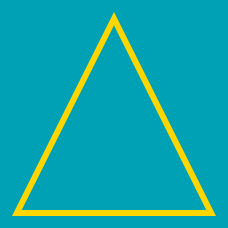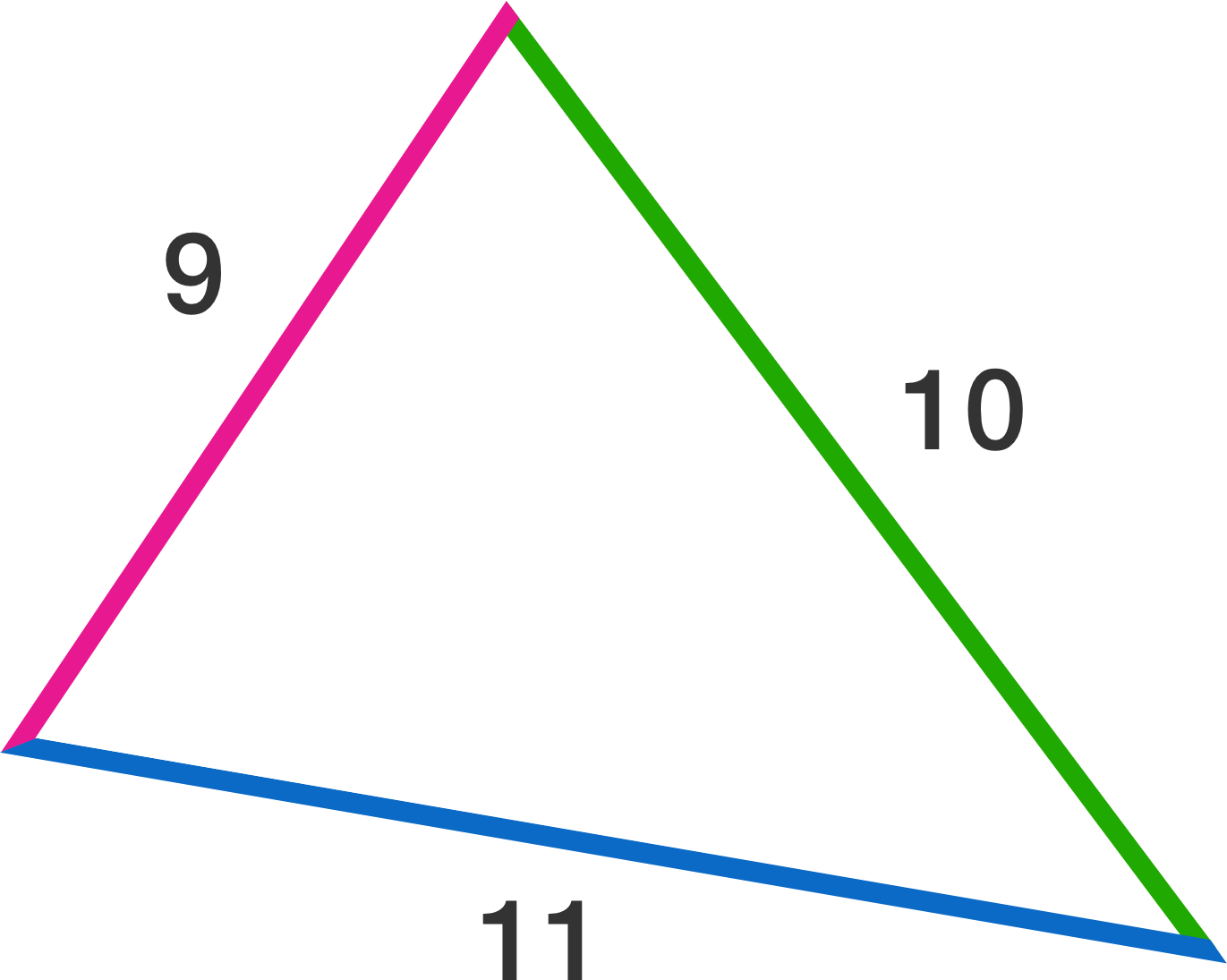Geometry

# Area of Triangles - Heron's Formula

What is the area of a triangle with side lengths $$9,12,$$ and $$15?$$

What is the area of a triangle with side lengths $$13, 14,$$ and $$15?$$

What is the area of a triangle with side lengths $$9, 11,$$ and $$10?$$A triangle has perimeter 14 and area $$2\sqrt{14}.$$ If the shortest side has length 3, find the positive difference between the lengths of other two sides. Give your answer to 3 decimal places.

What is the area of a triangle with side lengths $$3,5,$$ and $$7?$$

×

Problem Loading...

Note Loading...

Set Loading...